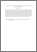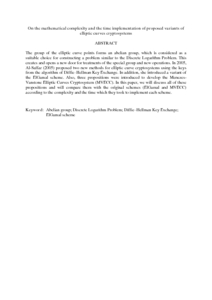Simple Search:

On the mathematical complexity and the time implementation of proposed variants of elliptic curves cryptosystems

Citation

Al-Saffar, Najlae F. Hameed and Md. Said, Mohamad Rushdan (2013) On the mathematical complexity and the time implementation of proposed variants of elliptic curves cryptosystems. International Journal of Cryptology Research, 4 (1). pp. 42-54. ISSN 1985-5753

Abstract / Synopsis

The group of the elliptic curve points forms an abelian group, which is considered as a suitable choice for constructing a problem similar to the Discrete Logarithm Problem. This creates and opens a new door for treatments of the special group and new operations. In 2005, Al-Saffar (2005) proposed two new methods for elliptic curve cryptosystems using the keys from the algorithm of Diffie–Hellman Key Exchange. In addition, she introduced a variant of the ElGamal scheme. Also, three propositions were introduced to develop the Menezes-Vanstone Elliptic Curves Cryptosystem (MVECC). In this paper, we will discuss all of these propositions and will compare them with the original schemes (ElGamal and MVECC) according to the complexity and the time which they took to implement each scheme.Preview
PDF (Abstract)
On the mathematical complexity and the time implementation of proposed variants of elliptic curves cryptosystems.pdfView Item